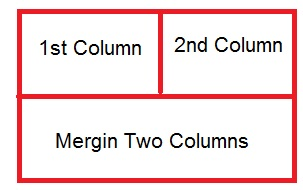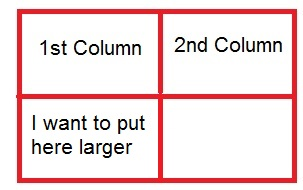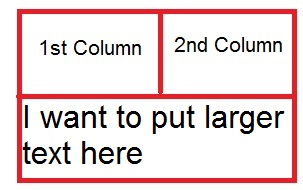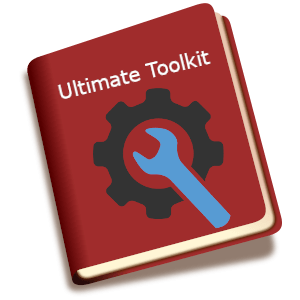# What is colspan? How to use colspan to merge column cells?

## What is colspan?

A colspan is the HTML attribute used in table header cells and data cells to merge columns

.

Merging is the same feature we use Microsoft Excel, in which We need to combine two or more than two columns. We use colspan in these cases.## How we use colspan in tables

Let us take a simple example to explain how to use colspan,

Let us consider, I want to use table contains 2 rows and two columns. In the first row of the table, I want to put data in the 1st colum and second one by one. I put data to the 1st row in 1st column, 1st row of 2nd column. Now in the 2nd row, I want to put only a single line larger length data. This data should not look very small and adjust to 1s and 2nd column of the table.

Here, If we put single data in the 2nd row without merging the two columns. We put it on the 2nd row and in the 1st column of the table like this:But if we do like this and put the data in the table cells. The one cell remain vacant in the table. It looks like the table is not arranged in the managed way.

Apply this example using HTML code:

Output

 PHP CSS These are the web development technical codes for combining table columns.

We need to merge the two columns to display the data in the managed way. In this way, I can merge the two columns using colspan attribute of the table. We need to use ‘colspan = 2’ to merge the 2 columns of the table. After I merge the columns the table will appear like this:In the above given image, we can check that, I have merged the two columns and put the text with the larger font size.

Let us see this with ‘colspan’ attribute using in the HTML code:

Output

 PHP CSS These are the web development technical codes for combining table columns.

If we want to merge the three columns of a table, we use colspan = “3”.

Similarly, if we want to merge four columns of a table, we use colspan=”4″ and so on.## THE ULTIMATE TOOLKIT

Get FREE Access to Toolkit and Resources that we are using in Tutorialdeep that Every Professional Should Have! in one handy PDF.